Next: Worked Examples Up: Inductance Previous: Transformers

## Impedance Matching

The principle use of transformers is in the transmission and distribution of commercially generated electricity. However, a second, very important use of transformers is as impedance matching devices. Recall, from Sect. 7.9, that for maximum power delivery from a source to a load, the load must have the same resistance as the internal resistance of the source. This can be accomplished by using a transformer to match the two resistances. Suppose that the power source is connected to the primary circuit, and the load to the secondary. If the resistance of the load is, then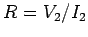. However, from the transformer equation, (299), we have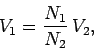(301)

and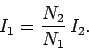(302)

Now the effective resistance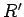of the load in the primary circuit is given by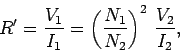(303)

which easily reduces to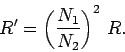(304)

Thus, by choosing the appropriate turns ratio, the effective load resistancecan be made equal to the internal resistance of the source, no matter what value the actual load resistancetakes. This process is called impedance matching.Next: Worked Examples Up: Inductance Previous: Transformers
Richard Fitzpatrick 2007-07-14